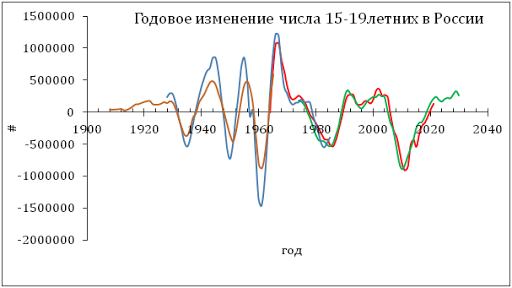## 1/27/12

### Two more graphs on GDP in the USA

Two more graphs on real GDP in the USA. In the forth quarter of 2011, the level of real GDP was higher (\$13,422 .4 billion) than that in the fourth quarter of 2007 (\$13,326 billion) as Figure 1 shows. Figure 2 demonstrates that  the increasing population did not allow real GDP per capita t reach the level of 2007: \$42,727 vs. \$43,791. In seems to be the task for 2012 to 2014 if no recession will occur.

Figure 1. Real GDP

Figure 2. Real GDP per capita